## Saturday, 9 August 2014

### chapter 10 Worked Out Examples

 Example: 1
Evaluate the following limits
 (a)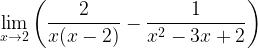$\mathop {\lim }\limits_{x \to 2} \left( {\dfrac{2}{{x(x - 2)}} - \dfrac{1}{{{x^2} - 3x + 2}}} \right)$ (b)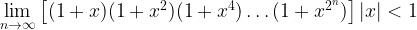$\mathop {\lim }\limits_{n \to \infty } \left[ {(1 + x)(1 + {x^2})(1 + {x^4})\ldots(1 + {x^{{2^n}}})} \right]|x|< 1$ (c)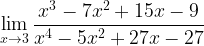$\mathop {\lim }\limits_{x \to 3} \dfrac{{{x^3} - 7{x^2} + 15x - 9}}{{{x^4} - 5{x^2} + 27x - 27}}$
 Solution: 1-(a)
This limit is of the indeterminate form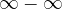$\infty - \infty$. Combining the two dfractions in this limit should lead to a cancellation of the factor giving rise to this indeterminacy, i.e.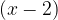$(x - 2)$$\mathop {\lim }\limits_{x \to 2} \left( {\dfrac{2}{{x(x - 2)}} - \dfrac{1}{{{x^2} - 3x + 2}}} \right)$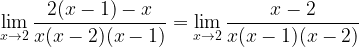$\mathop {\lim }\limits_{x \to 2} \dfrac{{2(x - 1) - x}}{{x(x - 2)(x - 1)}} = \mathop {\lim }\limits_{x \to 2} \dfrac{{x - 2}}{{x(x - 1)(x - 2)}}$ Cancelling out$(x - 2)$ from the numerator and the denominator.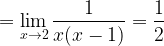$= \mathop {\lim }\limits_{x \to 2} \dfrac{1}{{x(x - 1)}} = \dfrac{1}{2}$
 Solution: 1-(b)
Before trying to solve this, try to feel that this expression will have a finite limit even though the number of factors being multiplied tends to infinity. This is because the successive factors become closer and closer to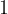$1$ and their ‘contribution’ to the final product becomes smaller and smaller Now, to simplify this product, we multiply it by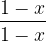$\dfrac{{1 - x}}{{1 - x}}$. This is what happens:$\mathop {\lim }\limits_{n \to \infty } \left[ {\dfrac{{\overbrace{(1 - x).(1 + x)}(1 + {x^2})(1 + {x^4})\ldots(1 + {x^{{2^n}}})}}{{(1 - x).}}} \right]$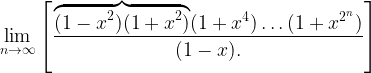$\mathop {\lim }\limits_{n \to \infty } \left[ {\dfrac{{\overbrace{(1 - {x^2})(1 + {x^2})}(1 + {x^4})\ldots (1 + {x^{{2^n}}})}}{{(1 - x).}}} \right]$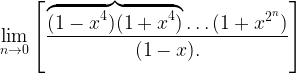$\mathop {\lim }\limits_{n \to 0} \left[ {\dfrac{{\overbrace{(1 - {x^4})(1 + {x^4})}\ldots (1 + {x^{{2^n}}})}}{{(1 - x).}}} \right]$$\vdots$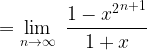$= \mathop {\lim }\limits_{n \to \infty } \,\,\dfrac{{1 - {x^2}^{n + 1}}}{{1 + x}}$
Since,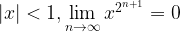$|x| < 1,\mathop {\lim }\limits_{n \to \infty } {x^{{2^{n + 1}}}} = 0$

Hence, the value of the limit is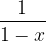$\dfrac{1}{{1 - x}}$
 Solution: 1-(c)
The numerator and denominator both tend to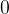$0$ as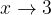$x \to 3$ because of the common factor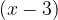$(x - 3)$.
Hence, factorization leads to :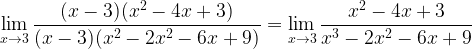$\mathop {\lim }\limits_{x \to 3} \dfrac{{(x - 3)({x^2} - 4x + 3)}}{{(x - 3)({x^2} - 2{x^2} - 6x + 9)}} = \mathop {\lim }\limits_{x \to 3} \dfrac{{{x^2} - 4x + 3}}{{{x^3} - 2{x^2} - 6x + 9}}$ Cancelling out$(x - 3)$ from both the numerator and the denominator There is still another common$(x - 3)$ left in both the numerator and the denominator.
Factorization again leads to
 Cancelling out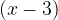$(x-3)$ again from the numerator and the denominator.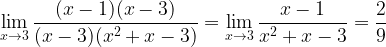$\mathop {\lim }\limits_{x \to 3} \dfrac{{(x - 1)(x - 3)}}{{(x - 3)({x^2} + x - 3)}} = \mathop {\lim }\limits_{x \to 3} \dfrac{{x - 1}}{{{x^2} + x - 3}} = \dfrac{2}{9}$
 Example: 2
Evaluate the following limits:
 (a)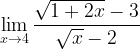$\mathop {\lim }\limits_{x \to 4} \dfrac{{\sqrt {1 + 2x} - 3}}{{\sqrt x - 2}}$ (b)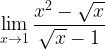$\mathop {\lim }\limits_{x \to 1} \dfrac{{{x^2} - \sqrt x }}{{\sqrt x - 1}}$ (c)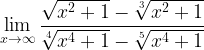$\mathop {\lim }\limits_{x \to \infty } \dfrac{{\sqrt {{x^2} + 1} - \sqrt{{{x^2} + 1}}}}{{\sqrt{{{x^4} + 1}} - \sqrt{{{x^4} + 1}}}}$ (d)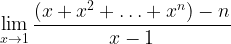$\mathop {\lim }\limits_{x \to 1} \dfrac{{(x + {x^2} +\ldots+ {x^n}) - n}}{{x - 1}}$
 Solution: 2-(a)
This limit can evidently be solved by rationalising both the numerator and the denominator.$\mathop {\lim }\limits_{x \to 4} \left\{ {\dfrac{{\sqrt {1 + 2x} - 3}}{{\sqrt x - 2}} \times \dfrac{{\sqrt {1 + 2x} + 3}}{{\sqrt {1 + 2x} + 3}} \times \dfrac{{\sqrt x + 2}}{{\sqrt x + 2}}} \right\}$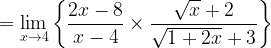$= \mathop {\lim }\limits_{x \to 4} \left\{ {\dfrac{{2x - 8}}{{x - 4}} \times \dfrac{{\sqrt x + 2}}{{\sqrt {1 + 2x} + 3}}} \right\}$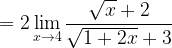$= 2\mathop {\lim }\limits_{x \to 4} \dfrac{{\sqrt x + 2}}{{\sqrt {1 + 2x} + 3}}$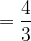$= \dfrac{4}{3}$
 Solution: 2-(b)
 This can be solved by rationalisation again.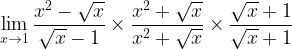$\mathop {\lim }\limits_{x \to 1} \dfrac{{{x^2} - \sqrt x }}{{\sqrt x - 1}} \times \dfrac{{{x^2} + \sqrt x }}{{{x^2} + \sqrt x }} \times \dfrac{{\sqrt x + 1}}{{\sqrt x + 1}}$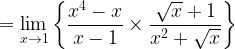$= \mathop {\lim }\limits_{x \to 1} \left\{ {\dfrac{{{x^4} - x}}{{x - 1}} \times \dfrac{{\sqrt x + 1}}{{{x^2} + \sqrt x }}} \right\}$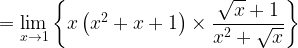$= \mathop {\lim }\limits_{x \to 1} \left\{ {x\left( {{x^2} + x + 1} \right) \times \dfrac{{\sqrt x + 1}}{{{x^2} + \sqrt x }}} \right\}$
 Solution: 2-(c)
This limit is of the indeterminate form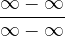$\dfrac{{\infty - \infty }}{{\infty - \infty }}$ (and look very complicated !)
However, division of both the numerator and denominator by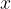$x$ directly reduces the limit to a determinate form.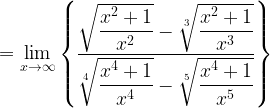$= \mathop {\lim }\limits_{x \to \infty } \left\{ {\dfrac{{\sqrt {\dfrac{{{x^2} + 1}}{{{x^2}}}} - \sqrt{{\dfrac{{{x^2} + 1}}{{{x^3}}}}}}}{{\sqrt{{\dfrac{{{x^4} + 1}}{{{x^4}}}}} - \sqrt{{\dfrac{{{x^4} + 1}}{{{x^5}}}}}}}} \right\}$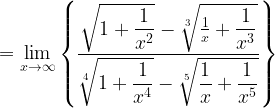$= \mathop {\lim }\limits_{x \to \infty } \left\{ {\dfrac{{\sqrt {1 + \dfrac{1}{{{x^2}}}} - \sqrt{{\frac{1}{x} + \dfrac{1}{{{x^3}}}}}}}{{\sqrt{{1 + \dfrac{1}{{{x^4}}}}} - \sqrt{{\dfrac{1}{x} + \dfrac{1}{{{x^5}}}}}}}} \right\}$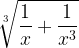${\sqrt{{\dfrac{1}{x} + \dfrac{1}{{{x^3}}}}}}$ and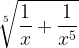${\sqrt{{\dfrac{1}{x} + \dfrac{1}{{{x^5}}}}}}$ these two terms tend to$0$ as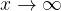$x \to \infty$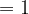$=1$
 Solution: 2-(d)
since the denominator is$x - 1$, we can get a hint that the numerator$(x + {x^2} + \ldots + {x^n}) - n$
can be written as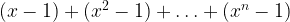$(x - 1) + ({x^2} - 1) +\ldots + ({x^n} - 1)$
so that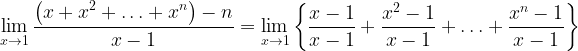$\mathop {\lim }\limits_{x \to 1} \dfrac{{\left( {x + {x^2} + \ldots+ {x^n}} \right) - n}}{{x - 1}} = \mathop {\lim }\limits_{x \to 1} \left\{ {\dfrac{{x - 1}}{{x - 1}} + \dfrac{{{x^2} - 1}}{{x - 1}} +\ldots + \dfrac{{{x^n} - 1}}{{x - 1}}} \right\}$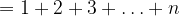$= 1 + 2 + 3 + \ldots + n$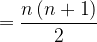$= \dfrac{{n\left( {n + 1} \right)}}{2}$